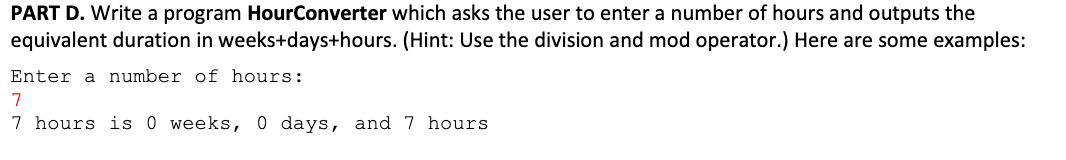# PART D. Write a program HourConverter which asks the user to enter a number of hours and outputs theequivalent duration in weeks+days+hours. (Hint: Use the division and mod operator.) Here are some examples:Enter a number of hours:7 hours is 0 weeks, 0 days, and 7 hours

Question
10 views

Not allowed to use decisions or loops, if then statements, I haven't learned that. I have to use division and modulus.help_outlineImage TranscriptionclosePART D. Write a program HourConverter which asks the user to enter a number of hours and outputs the equivalent duration in weeks+days+hours. (Hint: Use the division and mod operator.) Here are some examples: Enter a number of hours: 7 hours is 0 weeks, 0 days, and 7 hours fullscreen
check_circle

Step 1

Since here no programming language is specified. So, we are providing the solution in Java.

The below given java program will obey the following rubrics:

• Defining main method in main class.
• Creating instance of scanner class.
• Displaying message to user to enter the number of hours.
• Reading number of hours as input from user.
• For obtaining the number of hours, by using modulus operator. Modulus operator stores the completely divisible number only and ignores the remainder part.
• After that calculating the number of weeks by dividing the entered number of hours by 7 and storing the result in week variable.
• Finally, calculating the number of days by using the modulus operator in between the entered number of hours and the weeks.
• Displaying results to user.

Step 2

Program code:

import java.util.Scanner;

//main class

public class Main

{

//main method

public static void main(String[] args)

{

//instantiating scanner class

Scanner in = new Scanner(System.in);

//dispalying message to user to enter the number of hours

System.out.print("Enter a number of hours: \n");

int hours = in.nextInt();

//storing number of hours

int h1 = hours % 24;

//storing number of ...

### Want to see the full answer?

See Solution

#### Want to see this answer and more?

Solutions are written by subject experts who are available 24/7. Questions are typically answered within 1 hour.*

See Solution
*Response times may vary by subject and question.
Tagged in

### Computer Science